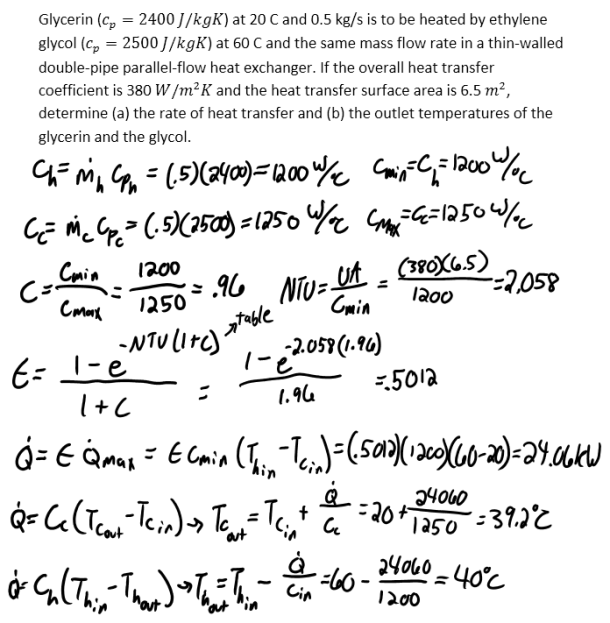heat and mass transfer problems engineering equations heat and mass transfer school homework engineering heat and mass transfer formulas heat and mass transfer solutions to heat and mass transfer problems full solution engineering problem solution heat and mass transfer math problems engineering equations heat and mass transfer school homework engineering solutions to heat and mass transfer formulas heat problems mass problem solutions to transfer problems full solution heat and mass transfer
heat and mass transfer problems engineering equations heat and mass transfer school homework engineering heat and mass transfer formulas heat and mass transfer solutions to heat and mass transfer problems full solution engineering problem solution heat and mass transfer math problems engineering equations heat and mass transfer school homework engineering solutions to heat and mass transfer formulas heat problems mass problem solutions to transfer problems full solution heat and mass transfer
Highalphabet Home Page heat and mass transfer problem solutions Heat and Mass Transfer Page
Glycerin (c_p=2400 J/kgK) at 20 C and 0.5 kg/s is to be heated by ethylene glycol (c_p=2500 J/kgK) at 60 C and the same mass flow rate in a thin-walled double-pipe parallel-flow heat exchanger. If the overall heat transfer coefficient is 380 W/m^2 K and the heat transfer surface area is 6.5 m^2, determine (a) the rate of heat transfer and (b) the outlet temperatures of the glycerin and the glycol.Glycerin (c_p=2400 J/kgK) at 20 C and 0.5 kg/s is to be heated by ethylene glycol (c_p=2500 J/kgK) at 60 C and the same mass flow rate in a thin-walled double-pipe parallel-flow heat exchanger. If the overall heat transfer coefficient is 380 W/m^2 K and the heat transfer surface area is 6.5 m^2, determine (a) the rate of heat transfer and (b) the outlet temperatures of the glycerin and the glycol.